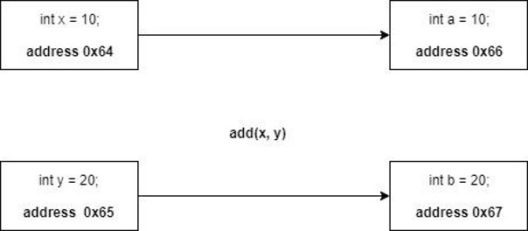# How to Implement Call by Reference in C++

Published on Sep 05,2019 64 ViewsWorking with C++ isn’t a difficult task if you already have a background in C as both of them share tons of concepts with each other. But, C++ has some concepts which aren’t a part of the C paradigm. In this Call by Reference in C++ article, we will talk about one such concept that is reference variables and its applications in the following order:

## Reference Variables

C++ gives us a new type of variable i.e a reference variable. We can think of a reference variable as a nickname for our original variable. That’s it that is the exact functionality of a reference variable in one line.

For example, if your name is Harrison but at home, your family members call you Harry. Now let’s go through some details of reference variables. The declaration and use of this type of variable are different from the usual variables we use but very much similar to the pointer variables.

Syntax:

```int Hello = 1;
int *ptr;
int &World = Hello;```

When we create a variable ‘Hello’ containing value ‘1’, a segment of memory is used to sore the value ‘1’ the name of this segment will be ‘Hello’ and the address of this segment will be some hex value for example 0x64. when we write `int &World = Hello; ` ‘World’ variable is created which is pointing towards the same memory location.

Including the line World++ will make changes in address 0x64 this means the value of variable ‘Hello’ will also change. Some might point out that how are reference variables different from the pointer variables which we will be discussing in the latter part of this post.

## Call by Value and Call by Reference in C++

Call by Value: Call by Value is a widely used method. Most of the times you will be using the call by value approach as you don’t want your original values of the variables to be changed. Hence we used the call by value method to call a function, only the values of the variables are passed. This is achieved by creating dummy variables in memory.

```#include <iostream>

using namespace std;

a = a + 10;
b = b + 10;
cout<<"Value of a = "<<a<<endl;
cout<<"Value of b = "<<b<<endl;

}

int main()
{
int x = 10;
int y = 20;

cout<<"Value of x = "<<x<<endl;
cout<<"Value of y = "<<y<<endl;

return 0;
}```

Output:

`Value of a = 20`

`Value of b = 30`

`Value of x = 10`

`Value of y = 20`From the above image, we can see that as soon as we call the add( ) function the values of x and y variables are copied to variables a and b. A and b are dummy variables.

Call by Reference: Now, let’s talk about the call by reference method. In this method separate dummy variables are not created, a reference of an already existing variable is passed to the method. This reference points to the same memory location hence separate copies are not made in the memory. The important point to note here is that the changes made in the reference variables are reflected in the dummy variable.

```#include <iostream>

using namespace std;

a = a + 10;
b = b + 10;
cout<<"Value of a = "<<a<<endl;
cout<<"Value of b = "<<b<<endl;

}

int main()
{
int x = 10;
int y = 20;

cout<<"Value of x = "<<x<<endl;
cout<<"Value of y = "<<y<<endl;

return 0;
}```

Output:

`Value of a = 20`

`Value of b = 30`

`Value of x = 20`

`Value of y = 30`

From the output, we can see that the original values of x and y are affected as separate dummy variables were not formed.## Return by Reference in C++

The way we can pass references to a function we can also return reference form a function.

```int & min(int &a, int &b)
{
if (a<b)
return a;

if (b<a)
return b;

}
```

As we can see that the above function looks different compared to normal functions since the return type is ‘int &’. This function returns a reference to a or b depending on the condition. The important point to note here is that the values are not returned.

If we call the function min(x, y) = 10. 10 will be assigned to x if it is minimum or to y if y is minimum.

## Call by Reference Vs Pointer

In all our examples we saw we always assigned a value to the reference variable we created since the reference variables cannot be NULL while pointer variables can be NULL and can cause unexpected errors.

Reference variables cannot be reassigned while pointer variables can point at some other variable later in the program.

A pointer variable holds the address value of a particular variable while the address of the reference variable is the same as that of the variable it is referring to.

With this, we come to an end of this Call By Reference in C++ article. I hope you got an understanding of the various implementation of the call by Value and reference and how they both differ.

If you wish to learn more, check out the Java Training by Edureka, a trusted online learning company. Edureka’s Java J2EE and SOA training and certification course is designed to train you for both core and advanced Java concepts along with various Java frameworks like Hibernate & Spring.

Got a question for us? Please mention it in the comments section of this blog and we will get back to you as soon as possible.